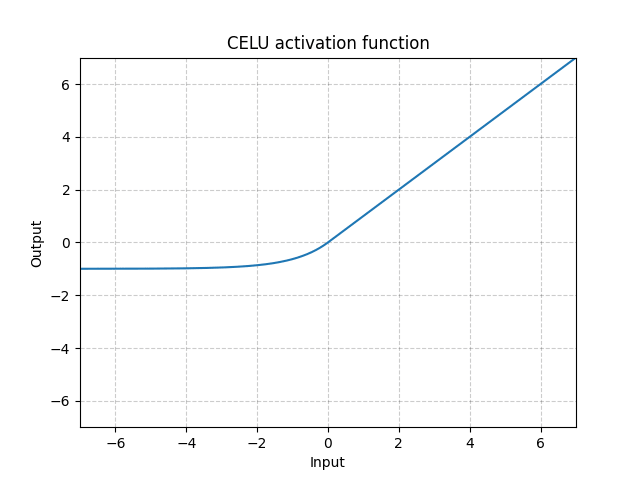/PyTorch

# CELU

class torch.nn.CELU(alpha: float = 1.0, inplace: bool = False) [source]

Applies the element-wise function:

$\text{CELU}(x) = \max(0,x) + \min(0, \alpha * (\exp(x/\alpha) - 1))$

More details can be found in the paper Continuously Differentiable Exponential Linear Units .

Parameters
• alpha – the $\alpha$ value for the CELU formulation. Default: 1.0
• inplace – can optionally do the operation in-place. Default: False
Shape:
• Input: $(N, *)$ where * means, any number of additional dimensions
• Output: $(N, *)$ , same shape as the inputExamples:

>>> m = nn.CELU()
>>> input = torch.randn(2)
>>> output = m(input)


© 2019 Torch Contributors
Licensed under the 3-clause BSD License.
https://pytorch.org/docs/1.7.0/generated/torch.nn.CELU.html